# Golden Ratio in Art and Architecture By Samuel ObaraGolden Ratio in Art and ArchitectureBy Samuel ObaraAccording to NCTM’s Principles and Standard for School Mathematics(2000), rich problems contexts involve connections to other disciplines, (e.g. science, social studies, art) as well as to the real world and to the daily life experience of middle-grades students(NCTM, 2000, p.374).
An exploration with the golden ratio offers opportunities to connect an understanding the conceptions of ratio and proportion to geometry. The mathematical connections between geometry and algebra can be highlighted by connecting Phi to the Fibonacci numbers and some golden figures.
Also, the golden ratio is a good topic to introduce historic and aesthetic elements to a mathematical concept, because we can find that not a few artists and architects were connected with the golden ratio of their works through much of the art history.

The one of purposes of this project is to overview the golden ratio briefly. The other is to introduce the occurrences of the golden ratio in art and architecture.

The content includes the following :

I.A discovery of the Golden Ratio
A. A brief history of the Golden Ratio
B. Definitions of the Golden Ratio related to Fibonacci sequence number
II. Some Golden Geometry
III. The Golden Ratio in Art and Architecture
IV. Resources
I. A discovery of Golden Ratio
A. A brief history of Golden Ratio

There are many different names for the golden ratio; The Golden Mean, Phi, the Divine Section, The Golden Cut, The Golden Proportion, The Divine Proportion, and tau(t).

The Great Pyramid of Giza built around 2560 BC is one of the earliest examples of the use of the golden ratio. The length of each side of the base is 756 feet, and the height is 481 feet. So, we can find that the ratio of the vase to height is 756/481=1.5717.. The Rhind Papyrus of about 1650 BC includes the solution to some problems about pyramids, but it does not mention anything about the golden ratio Phi.

Euclid (365BC – 300BC) in his “Elements” calls dividing a line at the 0.6180399.. point dividing a line in the extreme and mean ratio. This later gave rise to the name Golden Mean. He used this phrase to mean the ratio of the smaller part of this line, GB to the larger part AG (GB/AG) is the same as the ratio of the larger part, AG, to the whole line AB (AG/AB).Then the definition means that GB/AG = AG/AB.
proposition 30 in book VI

Plato, a Greek philosopher theorised about the Golden Ratio. He believed that if a line was divided into two unequal segments so that the smaller segment was related to the larger in the same way that the larger segment was related to the whole, what would result would be a special proportional relationship.

Luca Pacioli wrote a book called De Divina Proportione (The Divine Proportion) in 1509. It contains drawings made by Leonardo da Vinci of the 5 Platonic solids. Leonardo Da Vinci first called it the sectio aurea (Latin for the golden section).

Today, mathematicians also use the initial letter of the Greek Phidias who used the golden ratio in his sculptures.

B. Definitions of Golden Ratio

1) Numeric definition

Here is a ‘Fibonacci series’.

0, 1, 1, 2, 3, 5, 8, 13, 21, 34, 55, 89, 144, ..

If we take the ratio of two successive numbers in this series and divide each by the number before it, we will find the following series of numbers.

1/1 = 1
2/1 = 2
3/2 = 1.5
5/3 = 1.6666…
8/5 = 1.6
13/8 = 1.625
21/13 = 1.61538…
34/21 = 1.61904…

The ratio seems to be settling down to a particular value, which we call the golden ratio(Phi=1.618..).

2) Geometric definition

We can notice if we have a 1 by 1 square and add a square with side lengths equal to the length longer rectangle side, then what remains is another golden rectangle. This could go on forever. We can get bigger and bigger golden rectangles, adding off these big squares.

Step 2 Find the longer side
Step 3 Add another square of that side to whole thing

Here is the list we can get adding the square;
1 x 1, 2 x 1, 3 x 2, 5 x 3, 8 x 5, 13 x 8, 21 x 13, 34 x 21.
with each addition coming ever closer to multiplying by Phi.

start 1 by 1, add 1 by 1 => Now, it is 2 by 1, add 2 by 2Now, it is 3 by 2, add 3 by 3 => Now, it is 5 by 3, add 5 by 5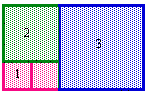Now, it is 8 by 5.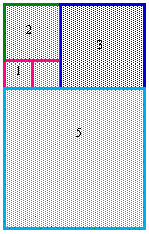3) Algebraic and Geometric definition

We can realize that Phi + 1 = Phi * Phi.Start with a golden rectangle with a short side one unit long.
Since the long side of a golden rectangle equals the short side multiplied by Phi, the long side of the new rectangle is 1*Phi = Phi.

If we swing the long side to make a new golden rectangle, the short side of the new rectangle is Phi and the long side is Phi * Phi.

We also know from simple geometry that the new long side equals the sum of the two sides of the original rectangle, or Phi + 1. (figure in page4)

Since these two expressions describe the same thing, they are equivalent, and so
Phi + 1 = Phi * Phi.

II. Some Golden Geometry

1) The Golden Rectangle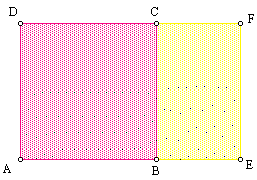A Golden Rectangle is a rectangle with proportions that are two consecutive numbers from the Fibonacci sequence.

The Golden Rectangle has been said to be one of the most visually satisfying of all
geometric forms. We can find many examples in art masterpieces such as in edifices of ancient Greece.

2) The Golden TriangleIf we rotate the shorter side through the base angle until it touches one of the legs, and then, from the endpoint, we draw a segment down to the opposite base vertex, the original isosceles triangle is split into two golden triangles. Aslo, we can find that the ratio of the area of the taller triangle to that of the smaller triangle is also 1.618. (=Phi)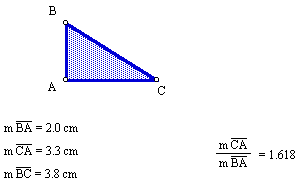If the golden rectangle is split into two triangles, they are called golden triangles suing the Pythagorean theorem, we can find the hypotenuse of the triangle.

3) The Golden SpiralThe Golden Spiral above is created by making adjacent squares of Fibonacci dimensions and is based on the pattern of squares that can be constructed with the golden rectangle.
If you take one point, and then a second point one-quarter of a turn away from it, the second point is Phi times farther from the center than the first point. The spiral increases by a factor of Phi.

This shape is found in many shells, particularly the nautilus.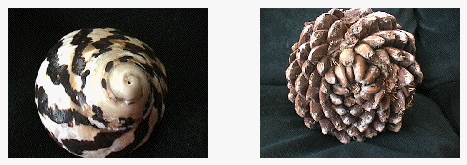4) Penrose Tilings

The British physicist and mathematician, Roger Penrose, has developed an aperiodic tiling which incorporates the golden section. The tiling is comprised of two rhombi, one with angles of 36 and 144 degrees (figure A, which is two Golden Triangles, base to base) and one with angles of 72 and 108 degrees (figure B).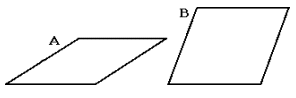When a plane is tiled according to Penrose’s directions, the ratio of tile A to tile B is the Golden Ratio.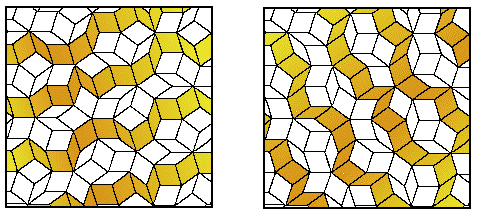In addition to the unusual symmetry, Penrose tilings reveal a pattern of overlapping decagons. Each tile within the pattern is contained within one of two types of decagons, and the ratio of the decagon populations is, of course, the ratio of the Golden Mean.

5) Pentagon and Pentagram

We can see there are lots of lines divided in the golden ratio. Such lines appear in the pentagon and the relationship between its sides and the diagonals.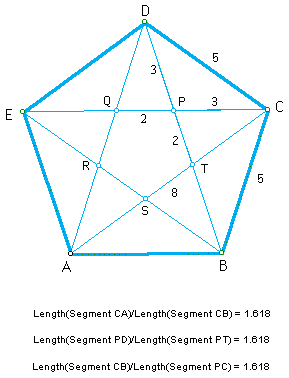We can get an approximate pentagon and pentagram using the Fibonacci numbers as lengths of lines. In above figure, there are the Fibonacci numbers; 2, 3, 5, 8. The ratio of these three pairs of consecutive Fibonacci numbers is roughly equal to the golden ratio.
III. Golden Ratio in Art and Architecture

A. Golden Ratio in Art

1) An Old man by Leonardo Da Vinci

Leonardo Da Vinci explored the human body involving in the ratios of the lengths of various body parts. He called this ratio the “divine proportion” and featured it in many of his paintings.Leonardo da Vinci’s drawing of an old man can be overlaid with a square subdivided into rectangles, some of which approximate Golden Rectangles.

2) The Vetruvian Man”(The Man in Action)” by Leonardo Da Vinci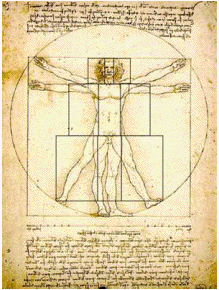We can draw many lines of the rectangles into this figure.
Then, there are three distinct sets of Golden Rectangles: Each one set for the head area, the torso, and the legs.

3) Mona-Risa by Leonardo Da VinciThis picture includes lots of Golden Rectangles. In above figure, we can draw a rectangle whose base extends from the woman’s right wrist to her left elbow and extend the rectangle vertically until it reaches the very top of her head. Then we will have a golden rectangle.
Also, if we draw squares inside this Golden Rectangle, we will discover that the edges of these new squares come to all the important focal points of the woman: her chin, her eye, her nose, and the upturned corner of her mysterious mouth.
It is believed that Leonardo, as a mathematician tried to incorporate of mathematics into art. This painting seems to be made purposefully line up with golden rectangle.

4) Holy Family by Micahelangelo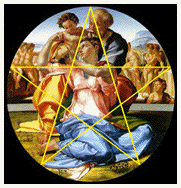We can notice that this picture is positioned to the principal figures in alignment with a Pentagram or Golden star.

5) Crucifixion by Raphaelhis picture is a well-known example, in which we can find a Golden Triangle and also Pentagram. In this picture, a golden triangle can be used to locate one of its underlying pentagrams.

6) self-portrait by RembrandtWe can draw three straight lines into this figure. Then, the image of the feature is included into a triangle. Moreover, if a perpendicular line would be dropped from the apex of the triangle to the base, the triangle would cut the base in Golden Section.

7) The sacrament of the Last Supper by Salvador Dali(1904-1989)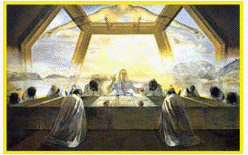This picture is painted inside a Golden Rectangle. Also, we can find part of an enormous dodecahedron above the table. Since the polyhedron consists of 12 regular Pentagons, it is closely connected to the golden section.

8) Golden Section Plate 1, 1993 by Fletcher Cox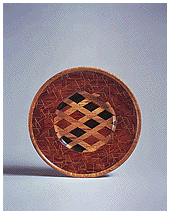The title of this work itself includes the Golden Section. It simply means that it is cut into sections of Golden Proportion.

9) Bathers by Seurat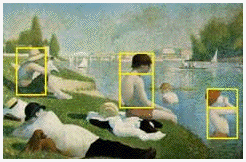Seurat attached most of canvas by the Golden Section. This picture has several golden subdivisions.
10) Composition with Gray and Light Brown by Piet Mondrian 1918

Mondrian believed that mathematics and art were closely connected. He used the simplest geometrical shapes and primary colours (blue, red, yellow).
His point of view lies in the fact that any shape is possible to create with basic geometric shapes as well as any color can be created with different combinations of red, blue, and yellow. The golden rectangle is one of the basic shapes appear in Mondrian’s art.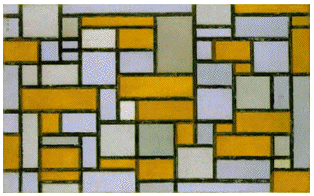Composition in Red, Yellow, and Blue(1926)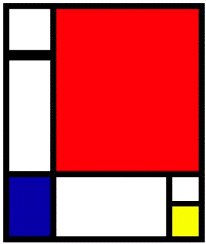We can find that the ratio of length to width for some rectangles is Phi.
B. Golden Ratio in Architecture

1) The Great Pyramid

The Ahmes papyrus of Egypt gives an account of the building of the Great Pyramid of Giaz in 4700 B.C. with proportions according to a “sacred ratio.”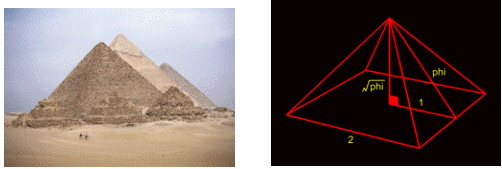2) Parthenon

The Greek sculptor Phidias sculpted many things including the bands of sculpture that run above the columns of the Parthenon.Even from the time of the Greeks, a rectangle whose sides are in the “golden proportion” has been known since it occurs naturally in some of the proportions of the Five Platonic. This rectangle is supposed to appear in many of the proportions of that famous ancient Greek temple in the Acropolis in Athens, Greece.

3) Porch of Maidens, Acropolis, Athens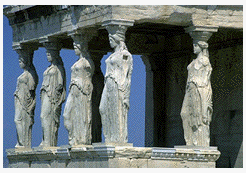4) Chartres CathedralThe Medieval builders of churches and cathedrals approached the design of their buildings in much the same way as the Greeks. They tried to connect geometry and art.
Inside and out, their building were intricate construction based on the golden section.

5) Le Corbussier

In 1950, the architect Le Corbussier published a book entitled “Le modulator. Essai
sur une mesure harmonique a l’echelle humaine applicable universalement a l’architecture et a la mecanique “. He invented the word “modulator” by combining “modul” (ratio) and “or” (gold); another expression for the well-known golden ratio.III. Resoureces

Internet

Michael’s Crazy Enterprises, Inc., The Golden Mean
(http://www.vashti.net/mceinc/)

The Golden Ratio
(http://www.math.csusb.edu/course/m128/golden/)

Ron Knott, The Golden section ratio : Phi
(http://www.ee.surrey.ac.uk/Personal/R.Knott/)

The Golden Ratio
(http://library.thinkquest.org/C005c449/)

Ron Knott, Fibonacci Numbers and Nature-part 2, Why is the Golden section the “best” arrangement?
(http://www.mcs.surrey.ac.uk/Personal/R.Knott/Fibonacci/)

Ron Knott, The Golden Section in Art, Architecture and Music
(http://www.mcs.surrey.ac.uk/Personal/R.Knott/Fibonacci/)

Steve Blacker, Jeantte Polanski, and Marc Schwach, The Golden Ratio
(http://www.geom.umn.edu/~demo5337/s97b/)

Ethan, The relations of the Golden ratio and the Fibonacci Series
(http://mathforum.org/dr.math/problems)

Golden Section in Art and Architecture
(http://www.camosun.bc.ca/~jbritton/goldslide/)

Sheri Davis and Danny Rhee, Mathematical Aspects of Arichitecture
(http://www.ma.uyexas.edu/~lefcourt/SP97/M302/projects/lefc023/)

Mathematics and Art
(http://www.q-net.au/~lolita/)

Leonardo da Vinci
(http://libray.thinkquest.org/27890/)

Math & Art : The golden Rectangle
(http://educ.queensu.ca/~fmc/october2001/)

Sue Meredith, Some Explorations with the Golden Ratio
( http://jwilson.coe.uga.edu/EMT668/)

What is a Fractal?
(http://ecsd2.re50j.k12.co.us/ECSD/)

Ron Knott, Phi’s Fascinating Figures
(http://www.euler.slu.edu/teachmaterial/)

Cynthia Lanius, Golden ratio Algebra
(http://math.rice.edu/~lanius/)

(http://www.exploremath.com/news/

Some Golden Geometry
(http://galaxy.cau.edu/tsmith/KW/)

Book
Robert L. (1989). Scared goemetry: philosophy and practice, New York: Thames
and Hudson.

Article

Donald, T. S. (1986). The Geometric Figure Relating the Golden Ratio and Phi,
Mathematics Teacher 79, 340-341.

Edwin, M. D. (1993). The Golden Ratio: A good opportunity to investigate multiple
representations of a problem, Mathematics Teacher 86, 554-557.

Susan, M. P. (1982). The Golden Ratio in Geometry, E. M. Maletsky, C. Hirsch, & D.
Yates(Eds.), Mathematics Teacher 75, 672-676.

This site uses Akismet to reduce spam. Learn how your comment data is processed.